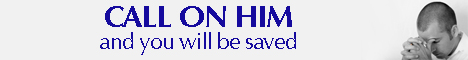Note:  Do not rely on this information. It is very old.

# Hyperbola

Hyperbola, in geometry, is one of the conic sections. It is the curve produced by the section of an ordinary right circular cone at any angle to the axis less than that at which the slant edges of the cone cut the axis. Its definition in analytical geometry is that it is the curve every point on which is at a distance from a given point that is a constant multiple of its distance from a given -straight line, this multiple being greater than unity. The curve has two identical branches, and extends to infinity in two directions. It has two axes of perfect symmetry, their intersection therefore constituting'the centre of the hyperbola. The above-mentioned fixed point and fixed line in the definition are called the focus and the directrix respectively. In every hyperbola there are two foci and two directrices, a pair of each, either of which pairs may be employed to develop the curve. The centre (q.v.) of the hyperbola, differs from that of a circle or an ellipse in that tangents may be drawn from it to the curve. Nevertheless, these tangents only touch the curve at infinite distance; they He in two lines symmetrically arranged about the axes, and are called asymptotes (q.v. see figure). The fundamental metrical property of the hyperbola may be shown to have the following rendering: the product of the distances of any point on the curve to the two asymptotes, when the distances are measured parallel to these two lines, will be always the same.